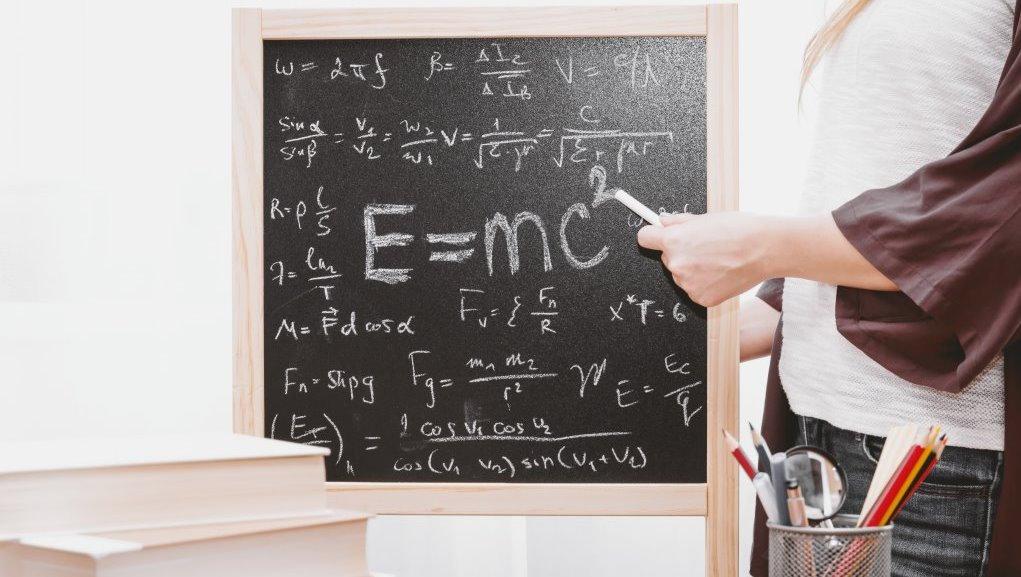# How to study Mathematics for competitive exams?

Mathematics is one of the scoring subjects in competitive exams. If you are pro in mathematics you can easily earn flying scores and get enrolled in top universities of your dreams. However, it is important for aspirants to learn shortcut tricks to do mathematical problems. Prior to taking the competitive exam, you need to test your abilities by taking online mathematics quizzes. This will let you know the points where you would need to improvise. Mathematics is definitely the scoring area and also the determining part of whether you can top the merit list or not. There are multiple sections in competitive exams in which you can score high. Undoubtedly, scoring in mathematics would eventually increase your total score. In addition to strategies, you also need to prepare for this exam stringently.

Every year, many aspirants will take the competitive exam and find mathematics as the most elusive part in the exam. You need to study and practice hard to gain a grip on the subject. Once you master the tricks of solving problems, you can easily earn high scores. The math would include algebra, probability, trigonometry, etc. It is not as easy as it looks, but with practice, you can get ready to give any competitive exam. There are many formulas, one should remember in this subject, which is what makes it complicated.Few of the tips one can keep in mind to study mathematics for competitive exams including

Choose the right training institute: When you are preparing for competitive exams, you would need the assistance of experts to learn the tricks which you can apply while solving problems in a matter of a few seconds. For this, you would need to get enrolled in a training institute no matter whether it is offline or online tutoring. This is the place where you can prepare and study well.

Manage time: You need to have good time management skills. You need to spend a lot of time on sharpening your skills on tough and complicated topics in mathematics. You need to take regular breaks while studying for competitive exams so that you would not get bored. In fact, you would come back with double energy to study.

Practice: You cannot study mathematics. All you need to do is to take a pen and paper and start solving the problems. The more you practice, the more quickly you would be able to solve simple to complicated problems. Every question has its own challenges. So, you need to try various ways to solve it, and find the right trick that you can apply to solve the similar kind of problems quickly.

Prepare the list of formulas: You need to note down the shortcuts and formulas on the paper and list out the formula that is used to solve multiple questions in the paper. You should note and remember the formulas; because the solutions would be based on the formula you apply. You would need to practice problems by applying formulas to remember them easily and solve problems with accurate answers.

Relate mathematics with real-time problems: When you use the approach of relating mathematical formulas to solve real-time problems, it lets you look it from a practice perspective.